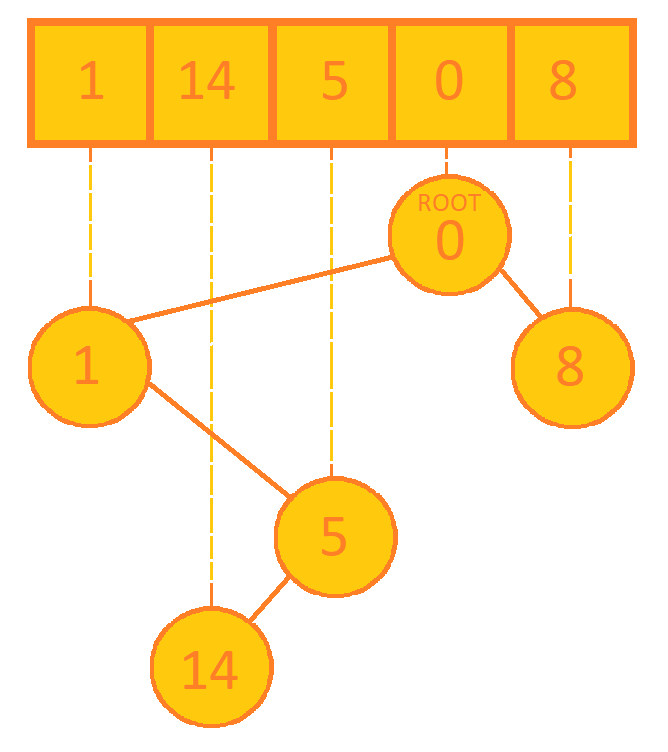×

Search anything:

# Cartesian Tree

#### Data Structures cartesian tree tree data structure Problems on Binary TreeIndian Technical Authorship Contest starts on 1st July 2023. Stay tuned.

Reading time: 20 minutes | Coding time: 10 minutes

A Cartesian tree is a binary rooted tree data structure that can answer range queries can be answered by finding least common ancestors in the tree. It follows following properties:

• The Cartesian tree formed from a sequence has a node for each number in that sequence.
• An inorder traversal of the tree would give the original sequence used to form the tree.
• Follows the heap property, i.e. each node has value greater than its parent (min heap) or each node has value smaller than its parent (max heap).

Cartesian tree is generally used as binary search tree for an ordered sequence and for answering range based queries. Cartesian tree has an important property that range queries can be answered by finding least common ancestors in the tree. Cartesian tree is not height balanced. The more common used variation of Cartesian tree is a Treap which assigns different priority to keys to make the tree balanced.

### Algorithm

#### Building the Cartesian tree

Below is linear algorithm to build Cartesian tree following min heap property.

1. Traverse the sequence from left to right.
2. For every node x, repeat steps 3 to 5.
3. Start at the node representing value before x, call it y. Check all nodes in path
the path from y to root of the tree (including y) until you reach a node with
value less than x, say z.
4. If z is found, set the z's right child to be new node, and z's previous child
to be left child of x.
5. If z is not found, set x to be root and set x's left child to be previous tree.

To create a tree with max heap property, in step 3, search for the first node
with value greater than x.

The Cartesian tree for the sequence {1, 14, 5, 0, 8} will be:Going from the tree rooted at 0, inorder traversal will give back the original sequence.

### Complexity

The time and space complexity of Cartesian Tree are:

$\bf{Space \hspace{1mm }complexity:}\hspace{1mm} O(N)$

$\bf{Time\hspace{1mm }complexities:}$

• $\bf{Build:} \hspace{1mm} O(N)$

### Implementations

C++ 11

/*
* c++ 11 code to construct a Cartesian tree. The code also contains a fucntion
* for inorder traversal of the tree to show that the order is maintained.
*/

#include <iostream>
#include <vector>

struct Node{
int value;
Node *left, *right;
Node *parent;

Node(){
value = 0;
parent = NULL;
left = NULL;
right = NULL;
}
};

class CartesianTree{
private:
// last pointer to keep track of last node added
Node *root, *last;

Node * findLowestNode(Node *node, int x){
// if(node == NULL)    // i.e. first insertion
//     return NULL;

if(node->value < x)
return node;
else if(node->parent != NULL)
return findLowestNode(node->parent, x);
else
return NULL;
}

public:
Node * getRoot(){
return root;
}

Node *new_node = new Node;
new_node->value = x;
if(root == NULL){
last = new_node;
root = new_node;
return;
}
Node *z = findLowestNode(last, x);
if(z == NULL){
new_node->left = root;
root->parent = new_node;
root = new_node;
}
else{
new_node->left = z->right;
z->right = new_node;
new_node->parent = z;
}

last = new_node;
}

CartesianTree(std::vector<int> ar){
root = NULL;
last = NULL;
// Call addNode function for each element of the array
for(auto x : ar){
}
}

void InorderTraversal(Node *node){
// To print inorder traversal of the tree
if(node == NULL)
return;
InorderTraversal(node->left);
std::cout << node->value << ' ';
InorderTraversal(node->right);
}
};

int main(){
std::vector<int> ar = {1, 14, 5, 0, 8};

CartesianTree tree(ar);
tree.InorderTraversal(tree.getRoot());
std::cout << '\n';
}

### Applications

• Cartesian tree sorting is an adaptive sorting algorithm that gives linear time complexity in favourable cases.
• Cartesian tree is used to construct a suffix tree.